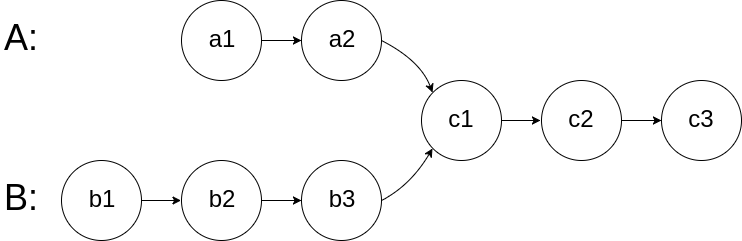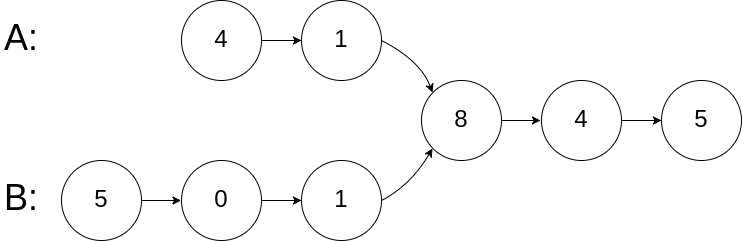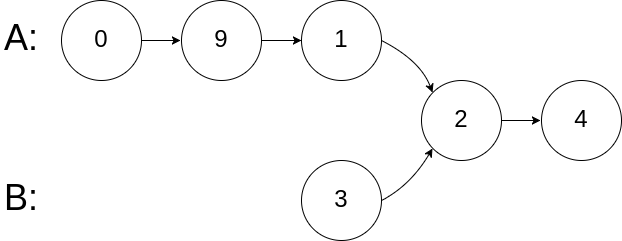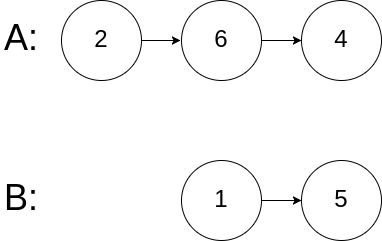# 【剑指Offer】双指针(1)

36 篇文章 0 订阅

#### 剑指 Offer 25. 合并两个排序的链表

输入：1->2->4, 1->3->4



0 <= 链表长度 <= 1000

/**
* struct ListNode {
*     int val;
*     ListNode *next;
*     ListNode(int x) : val(x), next(NULL) {}
* };
*/
class Solution {
public:
ListNode* mergeTwoLists(ListNode* l1, ListNode* l2) {
ListNode *p1 = l1, *p2 = l2, *head = nullptr, *p;
if(l1 == nullptr && l2 == nullptr)return head;
while(p1 && p2){
if(p1->val < p2->val){
p = p1;
p1 = p1->next;
}else{
p = p2;
p2 = p2->next;
}
}else{
if(p1->val < p2->val){
p->next = p1;
p = p1;
p1 = p1->next;
}else{
p->next = p2;
p = p2;
p2 = p2->next;
}
}
}
return p1 ? p1 : p2;
}
if(p1)p->next = p1;
if(p2)p->next = p2;
}
};#### 剑指 Offer 52. 两个链表的第一个公共节点输入：intersectVal = 8, listA = [4,1,8,4,5], listB = [5,0,1,8,4,5], skipA = 2, skipB = 3输入：intersectVal = 2, listA = [0,9,1,2,4], listB = [3,2,4], skipA = 3, skipB = 1输入：intersectVal = 0, listA = [2,6,4], listB = [1,5], skipA = 3, skipB = 2



• 如果两个链表没有交点，返回 null.
• 在返回结果后，两个链表仍须保持原有的结构。
• 可假定整个链表结构中没有循环。
• 程序尽量满足 O(n) 时间复杂度，且仅用 O(1) 内存。

/**
* struct ListNode {
*     int val;
*     ListNode *next;
*     ListNode(int x) : val(x), next(NULL) {}
* };
*/
class Solution {
public:
unordered_map<ListNode*, int> mp;
while(p != nullptr){
mp[p] = 1;
p = p->next;
}
while(p != nullptr){
if(mp.find(p) != mp.end()){
return p;
}
p = p->next;
}
return nullptr;
}
};/**
* struct ListNode {
*     int val;
*     ListNode *next;
*     ListNode(int x) : val(x), next(NULL) {}
* };
*/
class Solution {
public:
if(pA == nullptr || pB == nullptr)return nullptr;
while(pA != pB){
pA = pA != nullptr ? pA->next : headB;
pB = pB != nullptr ? pB->next : headA;
}
return pA;
}
};


// 相同长度
A: a1,a2,a3,a4,|c1|,c2
B: b1,b2,b3,b4,|c1|,c2

// 不同长度
A: a1,a2,a3,a4,c1,c2
B: b1,b2,c1,c2

pa:a1,a2,a3,a4,c1,c2,b1,b2,|c1|,c2
pb:b1,b2,c1,c2,a1,a2,a3,a4,|c1|,c209-214
02-21700
09-103872
11-19412
10-0946
04-2972
09-171101点击重新获取扫码支付余额充值